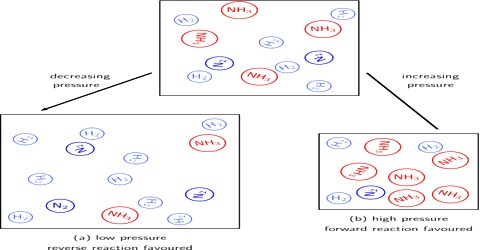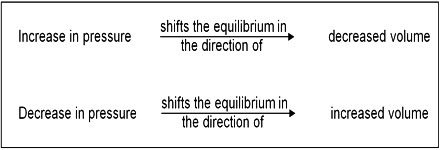# Effect of Pressure Change on Chemical Equilibrium

Effect of pressure change on chemical equilibrium

Homogeneous reactions in solution or in solid state are not accompanied by appreciable change in volume but this is not always the case with reactions involving gases. According to the principle of Le Chatelier, an increase of pressure on a reaction involving ago or gases should cause the equilibrium to shill in the direction which will result in a decrease in volume, because by doing so the effect of the pressure is diminished. By increase in pressure, the volume occupied by the system decreases. Hence the total number of moles per unit volume increases. To compensate this stress, the reaction which produces small number of moles of gaseous substance will be favored so that the increased pressure is reduced. For example:

N2 (g) + 3 H2 (g) ⇌ 2 NH3 (g)The decrease in volume takes place if, as a result of the reaction, the number of gaseous moles is decreased. However, in reactions where there is no volume change, i.e., there is no change in the number of mole; pressure has no effect on the equilibrium. These general remarks may be illustrated with the following example.

For the dissociation of phosphorus pentachloride into phosphorus trichloride and chlorine, the equilibrium constant in terms of the degree of dissociation is given by –

KP = [a2 / (1 – a2)] x P … … … (1)

If pressure P is increased, the denominator in equation (1) must increase in order to keep KP constant. This is possible, if the degree of dissociation decrease i.e., if some phosphorus trichloride and chlorine combine to form back phosphorus pentachloride. Conversely, if P is decreased reared, the degree of dissociation must increase so as to keep KP constant. For this reaction the expression for KP contains P as there is a change in the number of moles in the gaseous phase.